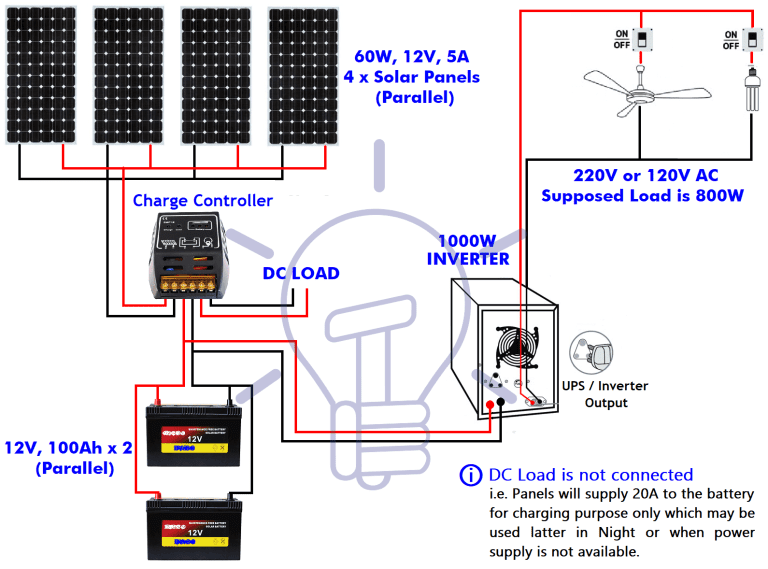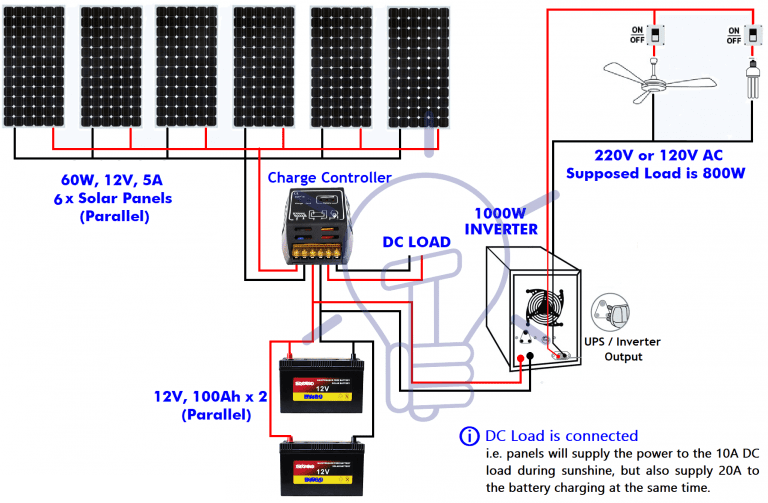JUBOLL Solar Power' Blog
This blog mainly focus on knowledge and industry news on Solar energy and its applications, ideas and FAQs.
By Mr. Liu | 12 May 2022 | 0 Comments

## A Complete Guide About Solar Power System Installation

Below is a DIY (do it yourself) complete note on Solar Power / Panel System installation, calculation about number of solar panels, batteries rating / backup time, inverter/UPS rating, load and required power in Watts.

Let's take 800W solar power system as an example:

We are going to install a solar power system in our home for a total load of 800W where the required backup time of battery is 3 hours.

Load = 800 Watts, Required Backup time for batteries = 3 Hours

1) Inverter / UPS Rating:

Inverter / UPS rating should be greater than 25% of the total load (for the future load as well as taking losses in consideration)

800 x (25/100) = 200W

Our Load + 25% Extra Power = 800+200 = 1000 Watts

This is the rating of the UPS (Inverter) i.e. We need 1000W UPS / Inverter for solar panel installation according to our need (based on calculations)

2）Quantity of batteries:
Now the required Back up time of batteries in Hours = 3 Hours

Suppose we are going to install 100Ah, 12 V batteries,

12V x 100Ah = 1200 Wh

Now for one battery (i.e. the Backup time of one battery)

1200 Wh / 800 W = 1.5 Hours

But our required Backup time is 3 Hours.

Therefore, 3/1.5 = 2 → i.e. we will have to connect two (2) batteries each of 100Ah, 12V. (connect 2 batteries in parallel)

3）Backup hours of batteries:

If the number of batteries are given, and you want to know the Backup Time for these given batteries, then use this formula to calculate the backup hours of batteries.

1200 Wh x 2 Batteries = 2400 Wh

2400 Wh / 800 W = 3 hours.

4) Charging current of batteries:

Charging current should be 1/10 of batteries Ah, that is:

200Ah x (1/10) = 20A

5) Charging time:
Here is the formula of Charging Time of a Lead acid battery.
Charging Time of battery = Battery Ah / Charging Current
T = Ah / A

For example, for a single 12V, 100Ah battery, The charging time would be:

T = Ah / A = 100Ah / 10A = 10 Hrs  (Ideal Case)

due to some losses, (it has been noted that 40% of losses occurred during the battery charging), this way, we take 10-12 A charging current instead of 10 A, this way, the charging time required for a 12V, 100Ah battery would be:

100Ah x ( 40/100 ) = 40 (100Ah x 40% of losses)

the battery rating would be 100Ah + 40 Ah = 140 Ah (100Ah + losses)

Now the required charging current for the battery would be:

140Ah / 12A = 11.6 Hours.

6) Required number of Solar Panels:

Scenario 1: DC Load is Not Connected = Only Battery Charging

We know the famous power formula (DC)

P = VI ………… (Power = Voltage x Current)

Putting the values of batteries and charging current.

P = 12V x 20 A

P = 240 Watts

these are the required wattage of solar panel (only for battery charging, and then battery will supply power to the load i.e. direct load is not connected to the solar panels)

Now

240W/60W = 4 pcs of Solar panels

Therefore, we will connect 4 Solar Panels (each of 60W,12V,5A) in parallel.The above calculations and system was only for battery charging (and then battery will supply power to the desired Load) to AC electrical appliances, which will get power through inverter and DC loads via Charge controller (via charged batteries)

Scenario 2: DC Load is Connected as well as Battery Charging

Now suppose there is a 10A directly connected load to the panels through inverter (or may be DC load via Charge Controller). During the sunshine, the solar panel provide 10A to the directly connected load + 20A to the battery charging i.e. solar panels charge the battery as well as provide 10A to the the load as well.

In this case, the total required current (20 A for Batteries Charging and 10 A for directly connected load)

In this case above, total required current in Amperes,

20A + 10 A = 30A

Now, I = 30 A, then required Power

P = V x I = 12V x 30A = 360 Watts

I.e. we need 360 W system for the above explained system (This is for both Direct Load and Batteries Charging)

Now, the number of solar panels we need

360/60W = 6 pcs of Solar Panels

Therefore, we will Connect 6 pcs of Solar panels in parallel (each of 60W, 12V,5A)7) Solar Controller:

As we have calculated above that the charging current for 200Ah battery is 20-22 Amperes (22A For Battery Charging+10A for direct DC Load), therefore we can use a charge controller about 30-32 Amp.

Note: The above calculation is based on ideal case, so it is recommended to always choose a solar panel some bigger then we need, because, there are some losses occurs during battery charging via solar panel as well as the sunshine is not always in ideal mood.

Now, with above knowledge, you can also build your own Solar Power Supply System, to power your lamps, fans, TV sets and other appliances.